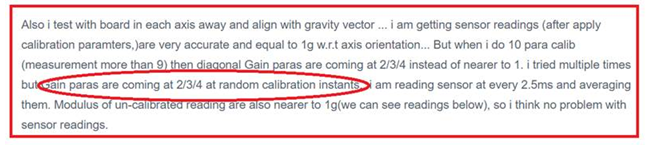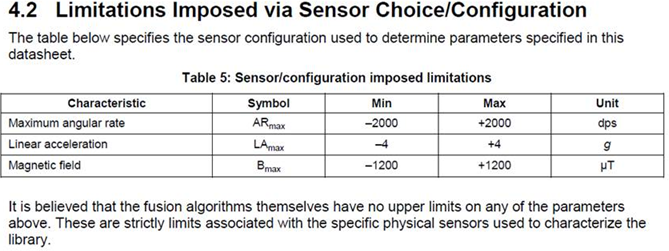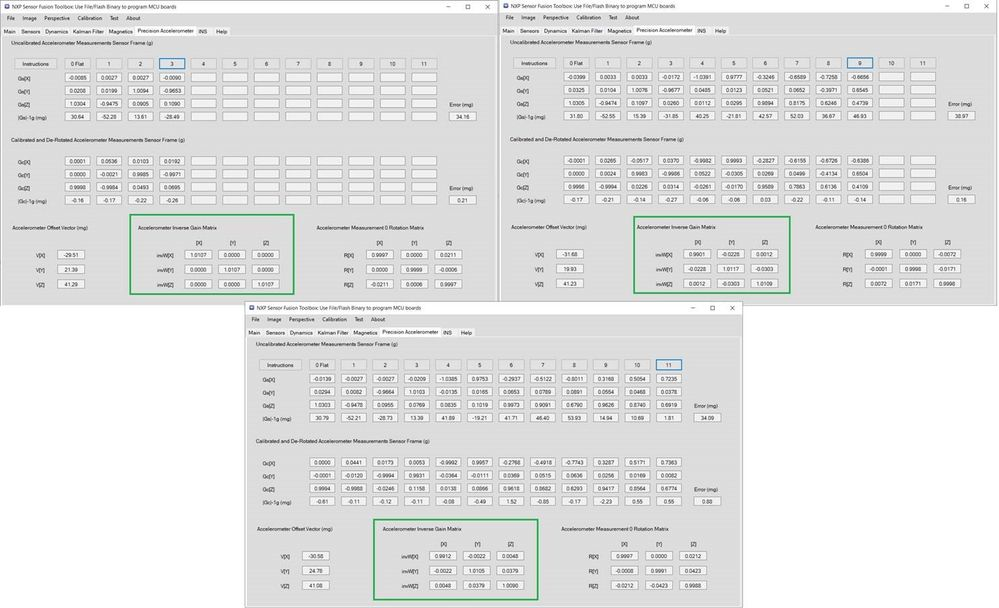## Problem with 10-para Precision Accelerometer Calibration of FXOS8700CQ

cancel
Showing results for
Did you mean:
SOLVED

## Problem with 10-para Precision Accelerometer Calibration of FXOS8700CQ

548 Views
Contributor II

We did buy FRDM_64F freedom board with 3 axis gyro, 3 axis accelerometer, 3 axis magnetometer. but USB not being detected on this board due to some problem as per my colleague and i cannot use it right now.

So i ported this code into DSP controller TMS320F28335. When i am doing precision accelerometer calibration as per AN5286 using 4 para, 7 para calibration, i feel i got the correct calibration gain parameters since, inverse Gain matrix diagonal values are nearer to 1 (but x,y,z offset para are very less 0.000xx).

Also i test with board in each axis away and align with gravity vector ... i am getting sensor readings (after apply calibration paramters,)are very accurate and equal to 1g w.r.t axis orientation... But when i do 10 para calib (measurement more than 9) then diagonal Gain paras are coming at 2/3/4 instead of nearer to 1. i tried multiple times but Gain paras are coming at 2/3/4 at random calibration instants.. i am reading sensor at every 2.5ms and averaging them. Modulus of un-calibrated reading are also nearer to 1g(we can see readings below), so i think no problem with sensor readings.

the changes i have done:

i am using XYZ data address at 0x33 (at 0x39 accelerometer reading comes) instead of 0x01 address so that magnetometer + accelerometer reading can be taken without changing the address inbetween (i2c address increment automatically.) and also i will get the directly right justified 16 bit data (no need to convert left shifted 16 bit data to 14 bit data). I changed setting 4g to 8g with sensitivity 1024. of course i tried with 4g setting (by correcting sensitivity to 2048(existing 8192) as i am using right justified accelerometer data) also but same problem with 10 para calibration.. diagonal gain parameters are no where nearer to 1.

I'm printing the below raw 12 averaged reading of accelerometer which i have used for calibration.. .

meas1 = (-0.0134082027, -0.0690478459, 0.966635764)

meas2 = (0.0177197251, 0.0200390629, -1.0054394)

meas3 = (-0.0132861324, -1.0000824213, -0.0650292933)

meas4 = (0.0158447269, 0.99924314, 0.028500976)

meas5 = (1.02742672, -0.0213916004, -0.0155908205)

meas6 = (-1.01027834, 0.00377929676, -0.0351611301)

meas7 = (-0.0197656248, -0.546865225, 0.811196268)

meas8 = (-0.0207958985, -0.745546877, 0.642041028)

meas9 = (-0.0193212889, -0.893725574, 0.433476567)

meas10 = (-0.00514160143, 0.446332991, 0.862988293)

meas11 = (-0.000698242162, 0.661806643, 0.719794929)

meas12 = (0.00429687509, 0.835830092, 0.523242176)

these readings are taken with 12 different orientation including standard each axis way and align gravity and other 6 reading with board +- 30, +- 45, +60 orientation. i have check the same algorithm in GNU Octave platform(similar to Matlab) and given above reading as input, then it showing an error that Inverse matrix elements are imaginary number where as offset parameters almost same as that of DSP software output.

So i really stuck with this problem...

So please anyone suggest me where i have gone wrong...

Also is it possible to check 10 parameters calib with above readings using FRDM_64F Freedom board or any uC board, to cross check the same problem is being repeated with above reading or not?

Labels (3)

• ### SensorFusion

1 Solution
412 ViewsNXP Employee

Hi Venkat,
The calibration algorithm performs a best fit of the measured data points to a 3D conic surface.

Usually this 3D surface shall be an ellipsoid but as your data set (i.e. sensor positions) is not quite well chosen, the algorithm actually yields another type of conic surface (paroboloid or hyperboloid), hence the surprising diagonal elements and complex numbers.

You can keep the first 6 orientations (faces of a cube) but the additional ones shall be more "equally" spread over all possibilities (of the 3D sphere). In your case, the X axis is about 0g for all last 6 positions, you simply need to select more diverse orientations as illustrated in the provided screen shots of the Sensor Fusion Toolbox GUI.

Regards,   Jacques.

6 Replies
412 Views
Contributor II

Thank you very much for your information.

When i calib for 4 parameters calibration(measurement >= 4), 7 parameters calibratation  (measurement >= 6) ... i found no issue since i am getting diagnal elements of Inverse Gain matrix are close to 1.

But when i extended measurement to >=9.. i am getting diagnal elements of inverse gain matrix are 2.x / 3.x etc...

Below are the measurement taken in sequence for 4,7,10 para calib...

meas1 = (-0.0134082027, -0.0690478459, 0.966635764)

meas2 = (0.0177197251, 0.0200390629, -1.0054394)

meas3 = (-0.0132861324, -1.0000824213, -0.0650292933)

meas4 = (0.0158447269, 0.99924314, 0.028500976) --- Execution of 4 para calib

meas5 = (1.02742672, -0.0213916004, -0.0155908205)  --- Execution of 4 para calib

meas6 = (-1.01027834, 0.00377929676, -0.0351611301)  --- Execution of 7 para calib

meas7 = (-0.0197656248, -0.546865225, 0.811196268) --- Execution of 7 para calib

meas8 = (-0.0207958985, -0.745546877, 0.642041028) --- Execution of 7 para calib

meas9 = (-0.0193212889, -0.893725574, 0.433476567) --- Execution of 10 para calib

meas10 = (-0.00514160143, 0.446332991, 0.862988293) --- Execution of 10 para calib

meas11 = (-0.000698242162, 0.661806643, 0.719794929) --- Execution of 10 para calib

meas12 = (0.00429687509, 0.835830092, 0.523242176) --- Execution of 10 para calib

Can you try 10 parameter calibration on  these above  measurements?  (may be editing the fGsStored[]  during debugging). Upto 8 measurments  i am getting gain elements of Inverse matrix close to one. But when i am taking 9th measurement the gain elements of Inverse matrix are 2.x /3.x randomly.

I feel there is no problem with sensor reading since Mod of these sensors reading close to 1. Am i right?

I implemented this 10 para calib algorithm in Octave (equivalent to Matlab) and applied above 12 measurements then it is generating complex number for Inverse gain matrix...

413 ViewsNXP Employee

Hi Venkat,
The calibration algorithm performs a best fit of the measured data points to a 3D conic surface.

Usually this 3D surface shall be an ellipsoid but as your data set (i.e. sensor positions) is not quite well chosen, the algorithm actually yields another type of conic surface (paroboloid or hyperboloid), hence the surprising diagonal elements and complex numbers.

You can keep the first 6 orientations (faces of a cube) but the additional ones shall be more "equally" spread over all possibilities (of the 3D sphere). In your case, the X axis is about 0g for all last 6 positions, you simply need to select more diverse orientations as illustrated in the provided screen shots of the Sensor Fusion Toolbox GUI.

Regards,   Jacques.

412 Views
Contributor II

Hi,Thank you so much for your reply and it helps me a lot.

I think your observation is correct and due to X axis near to zero in last measurements, the algorithm giving another type of conic surface (paroboloid or hyperboloid) instead of sphere.

first 6 readings i have taken as you mentioned... face of cube..... that means.. X axis away from gravity(+90) and aligned (-90 deg) gravity and repeated same for remaining Y,Z.

After that i couldn't read orientations illustrated in the provided screen shots of the Sensor Fusion Toolbox GUI so i have taken different orientation (Y axis -    30,45, 60, 120, 135, 150.).

Can you please suggest me the Orientation (X axis, Y axis, Z axis angles) to be kept for rest of 6 measurement.?

Regards,

Venkat.

412 ViewsNXP Employee

Hello,
Exact orientations are not critical (that's the benefit of the sphere fitting algorithm), they simply need to be significantly different from each others. Just add a mix of rotations (3 + 3) vs both X and Y axes.

412 ViewsNXP TechSupport

Hi,

Regarding the calibration, I will let amitpurohit‌ comment about it.

Best regards,

Tomas

412 ViewsNXP TechSupport

Hi,

I tried performing 12-point calibration multiple times using FRDM-K64F-AGM01 having FXOS8700 on Sensor Fusion toolbox and not able to create reported scenario (for quick reference providing below snapshot of issue reported):I would recommend checking the sensor register configuration used in your setup. Also see following sensor configuration limit as suggested by sensor fusion design spec document:Providing you below snapshot of my trials (with 4-point, 10-point, 12-point calibration) at my end (you can see that the acceleration inverse gain matrix diagonal values are always near to ~1).Best regards,

Tomas